# How to Calculate and Solve for Centripetal Acceleration, Angular Velocity and Radius of Circular Path of a Body | The Calculator Encyclopedia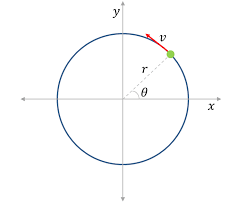The image above represents centripetal acceleration in a motion of circular path.

To compute for the centripetal acceleration, two essential parameters are needed and these parameters are angular velocity (ω) and radius of circular path (r).

The formula for the calculating centripetal acceleration:

a = ω²r

Where:
a = Centripetal Acceleration
ω = Angular Velocity
r = Radius of Circular Path

Let’s solve an example;
Find the centripetal acceleration with an angular velocity of 33 and a radius of 21.

This implies that;
ω = Angular Velocity = 33
r = Radius of Circular Path = 21

a = ω²r
a = 33² x 21
a = 1089 x 21
a = 22869

Therefore, the centripetal acceleration is 22869 m/s².

Calculating the Angular Velocity when Centripetal Acceleration and Radius of Circular path is Given.

ω = √a / r

Where:
ω = Angular Velocity
a = Centripetal Acceleration
r = Radius of Circular Path

Let’s solve an example;
Find the angular velocity with a centripetal acceleration of 320 and a radius of 8.

This implies that;
a = Centripetal Acceleration = 320
r = Radius of Circular Path = 8

ω = √a / r
ω = √320 / 8
ω = √40
ω = 6.32

Therefore, the angular velocity is 6.32.

Calculating the Radius of Circular path when Centripetal Acceleration and  Angular Velocity is Given.

r = a / ω2

Where:
r = Radius of Circular Path
a = Centripetal Acceleration
ω = Angular Velocity

Let’s solve an example;
Find the radius with a centripetal acceleration of 450 and an angular velocity of 15.

This implies that;
a = Centripetal Acceleration = 450
ω = Angular Velocity = 15

r = a / ω2
r = 450 / 152
r = 450 / 225
r = 2

Nickzom Calculator – The Calculator Encyclopedia is capable of calculating the centripetal acceleration.

To get the answer and workings of the centripetal acceleration using the Nickzom Calculator – The Calculator Encyclopedia. First, you need to obtain the app.

You can get this app via any of these means:

To get access to the professional version via web, you need to register and subscribe for NGN 1,500 per annum to have utter access to all functionalities.
You can also try the demo version via https://www.nickzom.org/calculator

Once, you have obtained the calculator encyclopedia app, proceed to the Calculator Map, then click on Mechanics under Engineering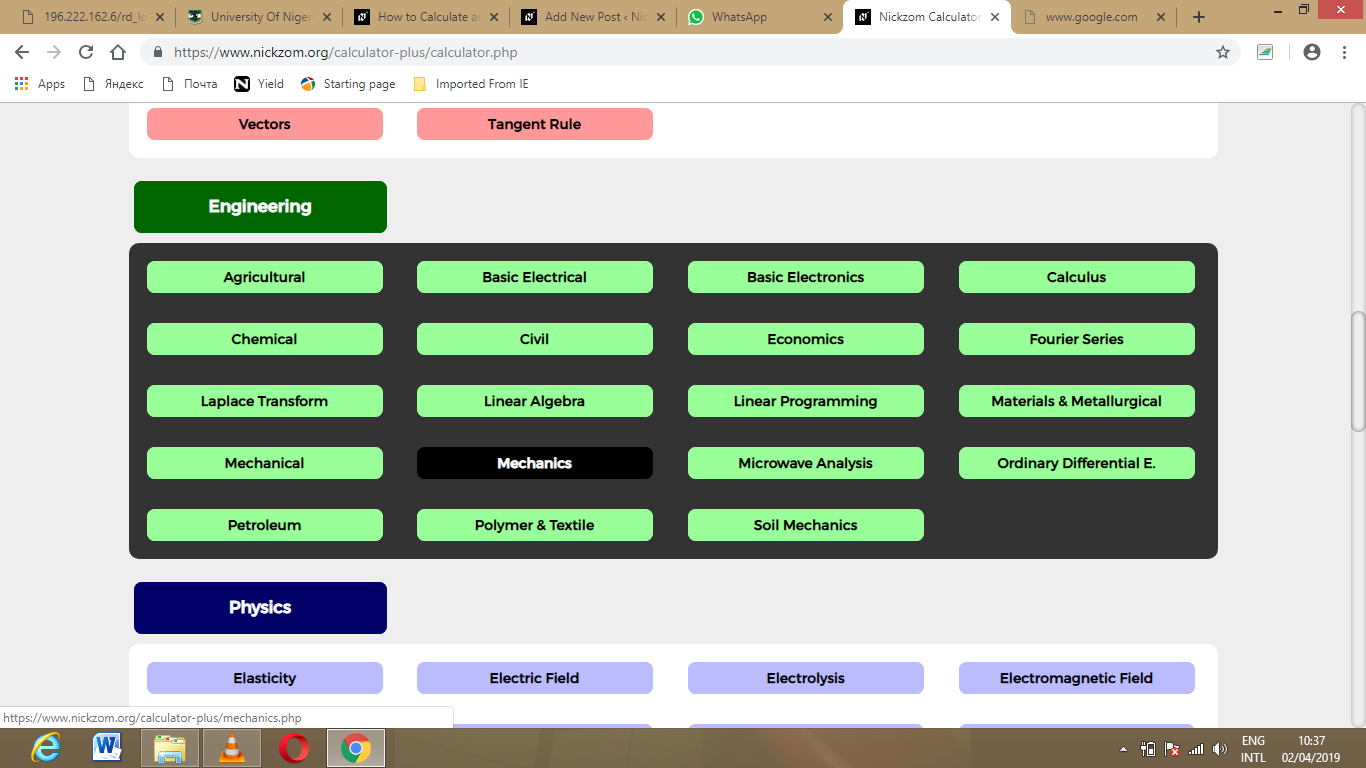Now, Click on Motion of Circular Path under Mechanics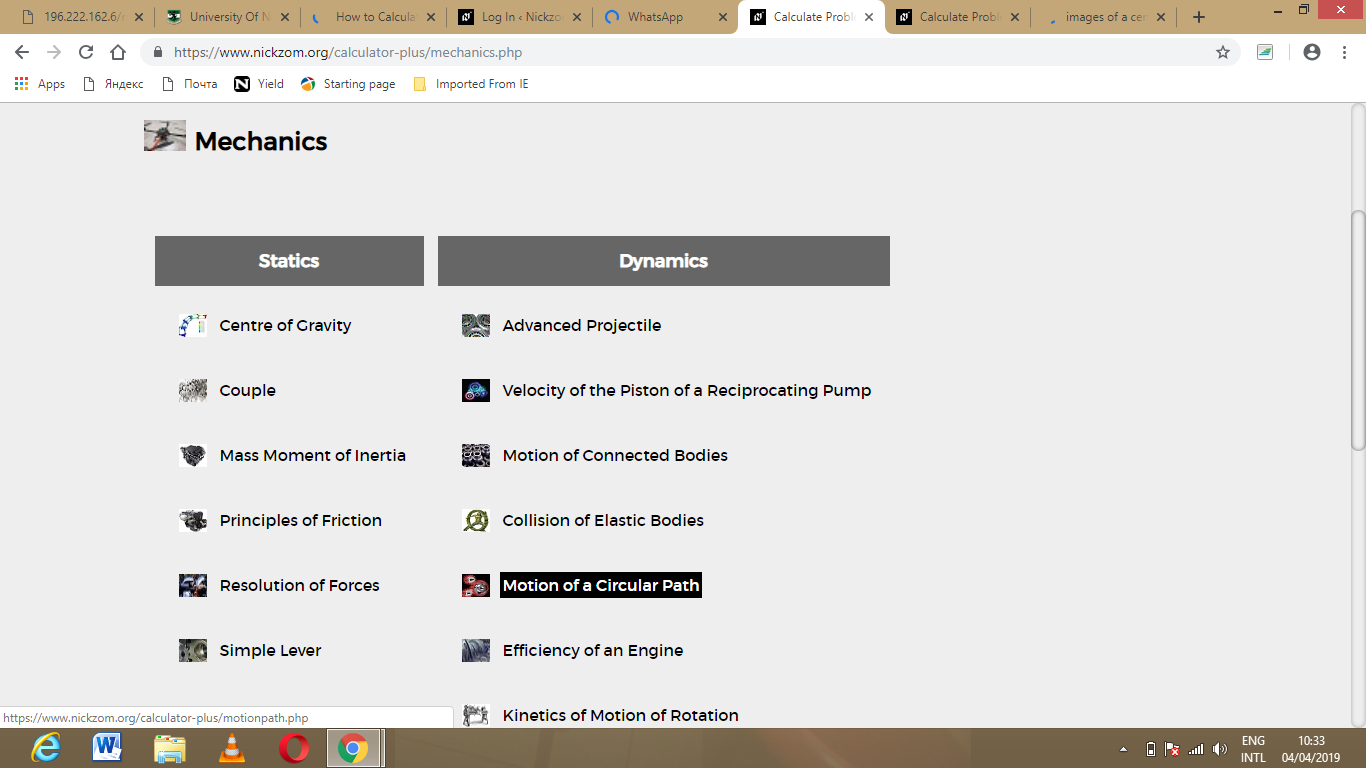Click on Centripetal Acceleration under Motion of Circular Path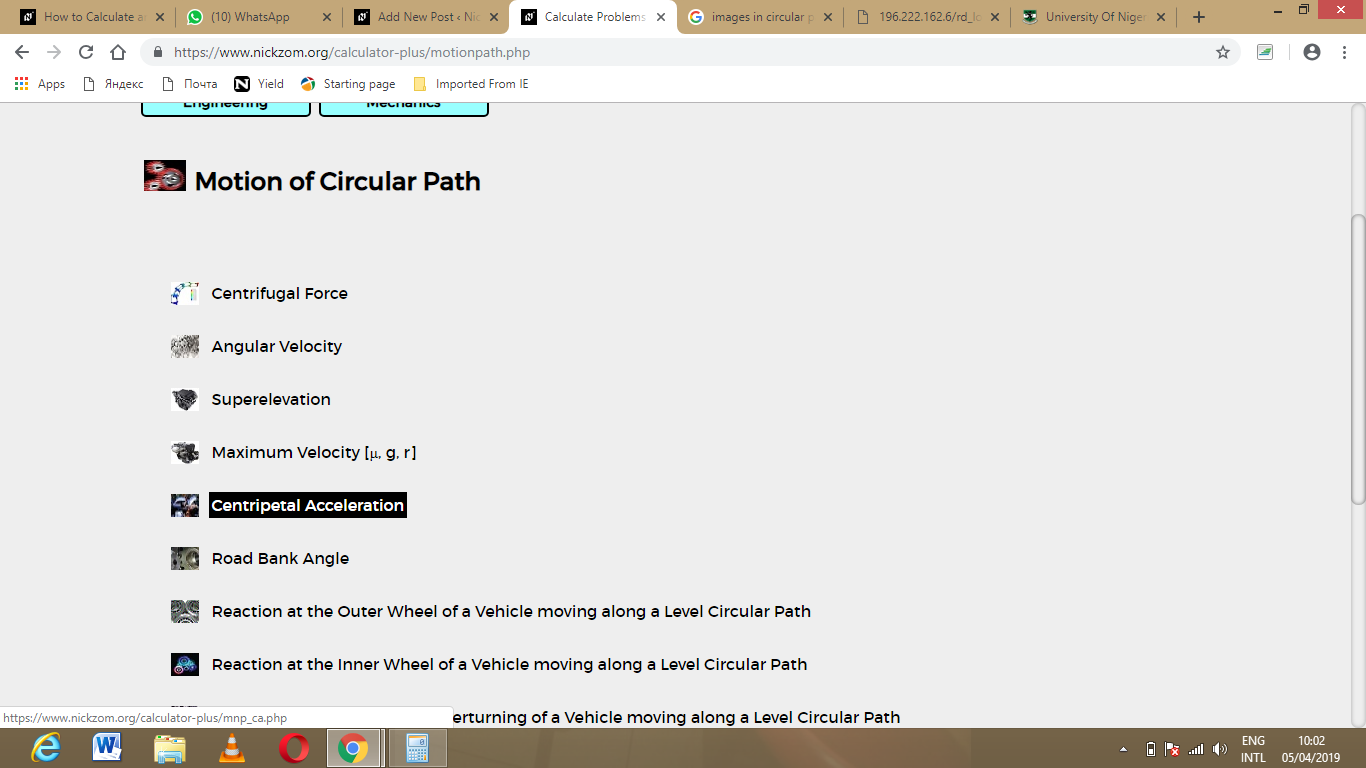The screenshot below displays the page or activity to enter your value, to get the answer for the centripetal acceleration according to the respective parameter which are the angular velocity (ω) and radius of circular path (r).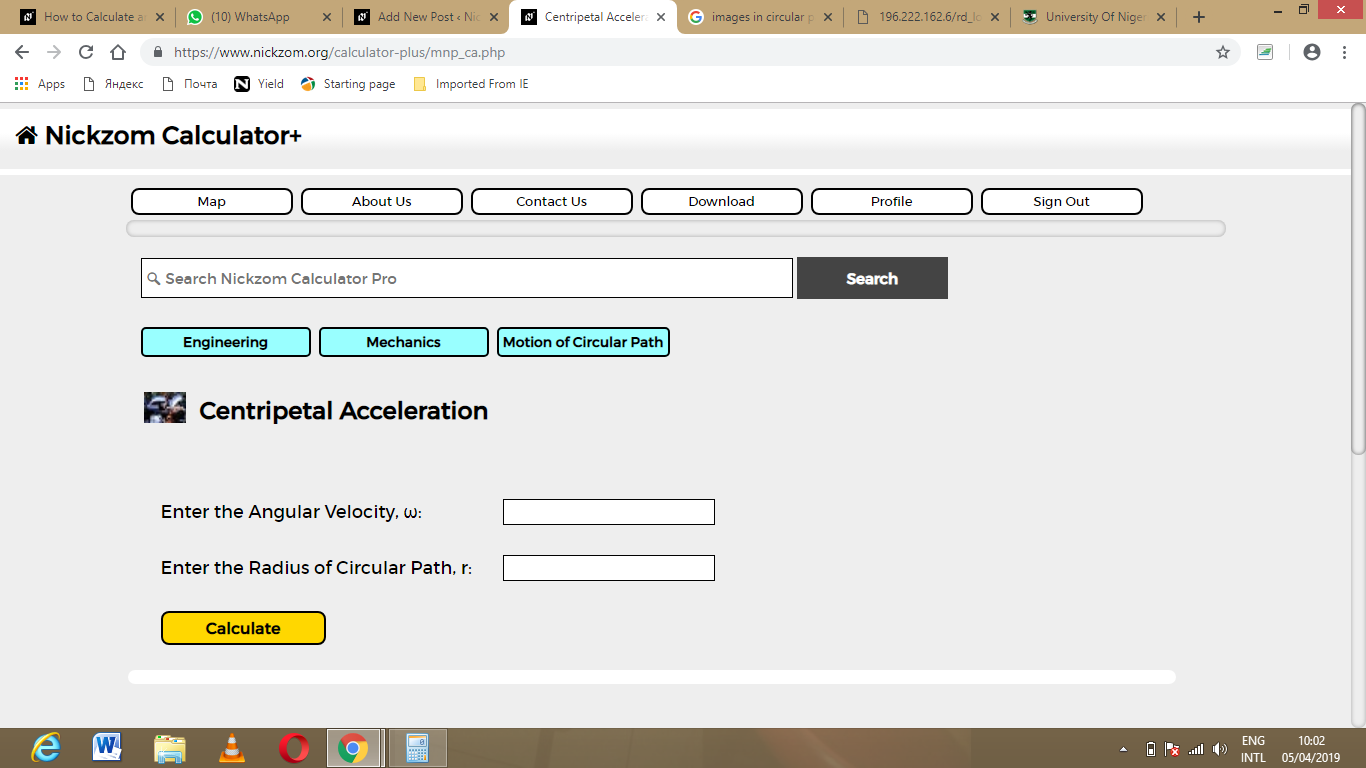Now, enter the value appropriately and accordingly for the parameter as required by the angular velocity (ω) is 33 and radius of circular path (r) is 21.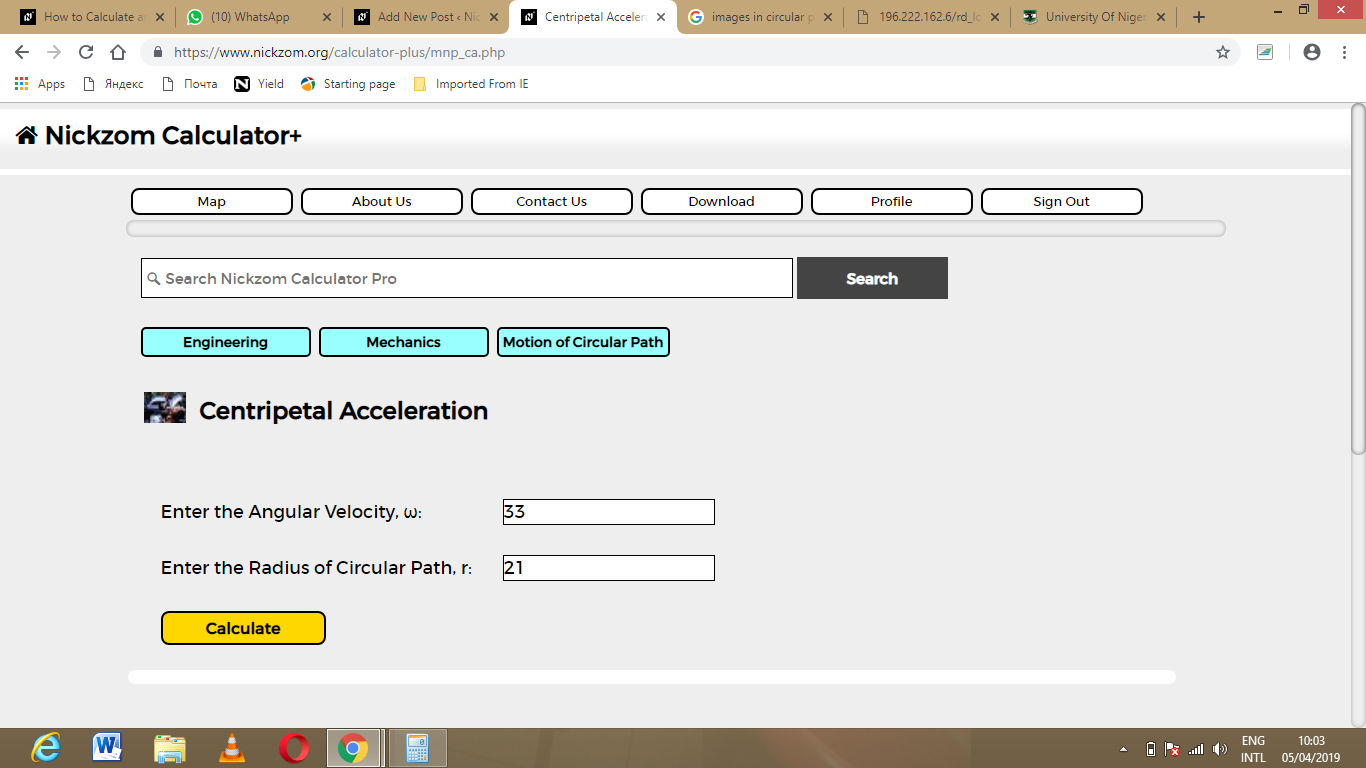Finally, Click on Calculate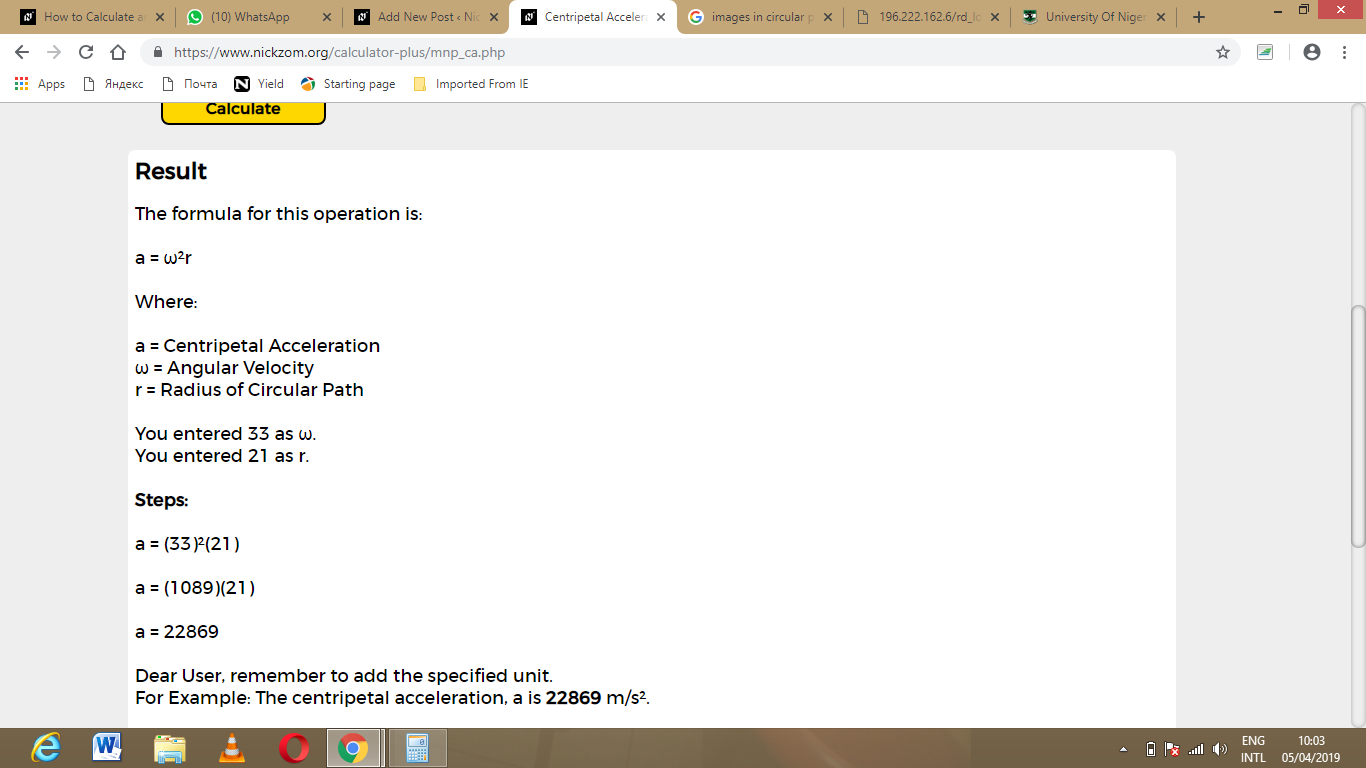As you can see from the screenshot above, Nickzom Calculator – The Calculator Encyclopedia solves for the centripetal acceleration and presents the formula, workings and steps too.